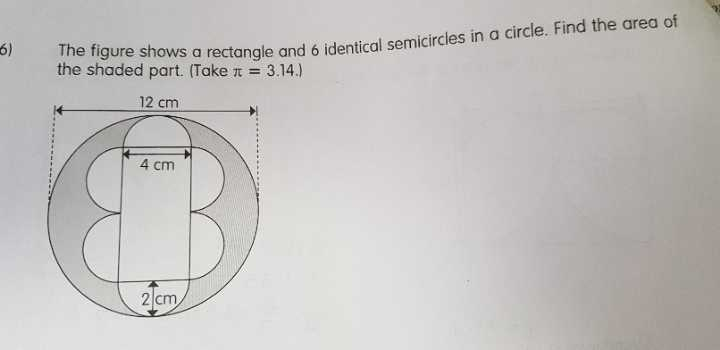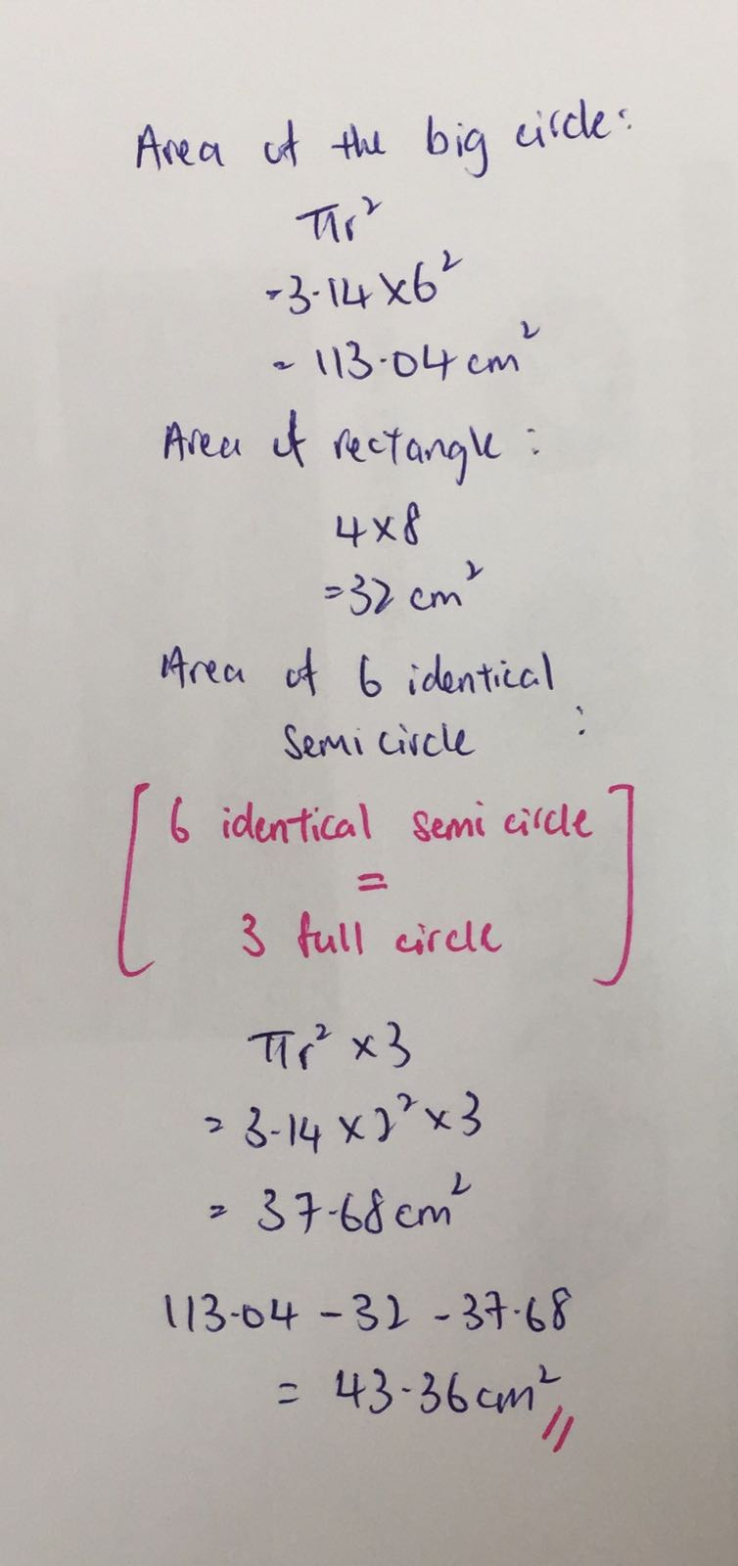# Question4 Answers

# Answer

area of the shaded part = (3.14 x 6 x 6) – (3.14 x 2 x 2 x 3) – [4 x (12 – 2 – 2)] = 43.36

Ans : 43.36 sq cm.

0 Replies 0 LikesHope this answers your question.

Owlinker
owlinker.com
FB Owlinker

0 Replies 0 Likes

Procedures to find the area of the shaded part:
1) Find the area of the large circle.
2) Find the area of the rectangle.
3) Find the area of the 6 semi-circles.
4) shaded area = area of large circle – area of 6 semi-circles – area of rectangle

Calculations:

Area of large circle: 3.14 x 6 x 6 = 113.04cm²
Area of rectangle: 4 x (4 + 4) = 4 x 8 = 32cm²
(Note: length of rectangle is equal to 2 x diameter of the small semi-circles)
Area of 6 semi-circles: (3.14 x 2 x 2) x 3 = 37.68cm²
(Note: 6 semi-circles is equal to 3 full circles)
Therefore, area of shaded part is 113.04 – 32 – 37.68 = 43.36cm²

0 Replies 0 Likes